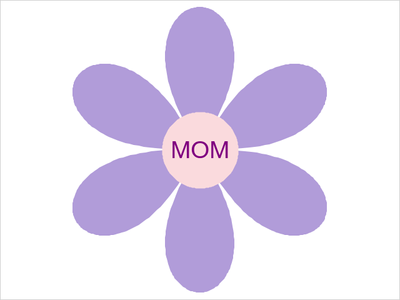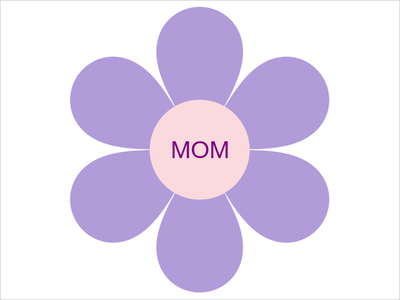## Fun With SAS ODS Graphics: (Polar) Flowers for Mother's DaySAS Polar FlowerCraft Project for Kids

What do you get when you cross @Rick_SAS's Polar Rose code with kids' crafts? Polar flowers for Mother's Day!

``````* Fun With SAS ODS Graphics: Mother's Day Polar Flower
References: blogs.sas.com/content/iml/2015/12/16/polar-rose.html
matrixlab-examples.com/polar-plots.html
thebestideasforkids.com/flower-template;

data flower;                               /* Generate points for "flower" */
id=1;
do theta = 0 to 2*constant("pi") by 0.01;
r = sqrt(abs(sin(3*theta)));             /* Six-petaled "flower" */
x = r*cos(theta);                        /* Convert to x/y */
y = r*sin(theta);
output;
end;
x=.; y=.; x2=0; y2=0; mom='MOM'; output;   /* Generate "pistil" */
/* Polygon/scatter/text plots */
proc sgplot data=flower aspect=1 noborder noautolegend;
polygon x=x y=y id=id / fill fillattrs=(color=cxb19cd9);
text x=x2 y=y2 text=mom / textattrs=(color=white size=28pt color=purple);
xaxis display=none min=-1 max=1 offsetmin=0 offsetmax=0;
yaxis display=none min=-1 max=1 offsetmin=0 offsetmax=0;``````
2 REPLIES 2Jay54
Meteorite | Level 14

## Re: Fun With SAS ODS Graphics: (Polar) Flowers for Mother's Day

Nice work TC.  I wonder if the same can be done using the ELLIPSEPARM statement(s).

## Re: Fun With SAS ODS Graphics: (Polar) Flowers for Mother's Day

You know, I forgot there was an ELLIPSEPARM statement for PROC SGPLOT! Gave it a quick shot, and it worked fine for generating a circle to replace the SCATTER plot, but I couldn't get a good shape for the petals. Also, I found that tweaking the equation for the petals yielded a more pleasing (to me at least!) shape for the petals:Take 2!

``````* Fun With SAS ODS Graphics: Mother's Day Polar Flower
References: blogs.sas.com/content/iml/2015/12/16/polar-rose.html
matrixlab-examples.com/polar-plots.html
thebestideasforkids.com/flower-template;

data flower;                               /* Generate points for "flower" */
id=1;
do theta = 0 to 2*constant("pi") by 0.01;
r = abs(sin(3*theta))**(1/4);            /* Six-petaled "flower" */
x = r*cos(theta);                        /* Convert to x/y */
y = r*sin(theta);
output;
end;
x=.; y=.; x2=0; y2=0; mom='MOM'; output;   /* Generate "pistil" */
/* Polygon/ellipse/text plots */
proc sgplot data=flower aspect=1 noborder noautolegend;
polygon x=x y=y id=id / fill fillattrs=(color=cxb19cd9);